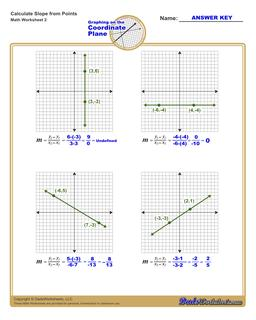PLEASE GO BACK AND USE THE BIG BLUE 'PRINT' BUTTON ON THE PAGE TO PRINT THE WORKSHEET CORRECTLY!Sorry for the trouble! The browser won't print the embedded worksheet PDF directly using the normal 'Print' command in the file menu, so you need to click the big 'Print' button to send just the worksheet and not the surrounding page to the printer.

Math Worksheets: Linear Equations: Linear Equations: Find the Slope of a Line (Second Worksheet)Find the Slope of a Line (Second Worksheet)

PropertyValue
DescriptionFind the Slope of a Line: The slope worksheets on this page require students to identify the direction of a the slope of a line, and also to find the slope from two points. (Second Worksheet)
Resource TypeWorksheet